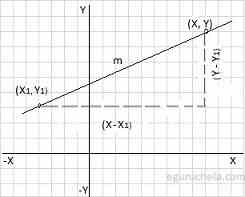# Point slope form Calculator

To calculate equation of a straight line, please enter the line coordinates (x1, y1) in the XY plane are used and Enter Slope Value (m).

X1 Y1

 Slope(m):

 Equation :

The point slope form is defined that the difference in the y-axis coordinate between two points (y - y1) on a line is proportional to the difference in the x-axis coordinate points (x - x1) and the proportionality constant m is the point slope of the straight line. The equation of a straight line can be found using following formula:

y – y1 = m(x – x1)Let's take an example where slop is 5 and coordinates (x, y) are (-6 , 8).
Now we can apply these values in given formula as follows:
y – y1 = m(x – x1)
=> y - 8 = 5(x - (-6))
=> y - 8 = 5(x + 6)
=> y - 8 = 5x + 30
=> 5x + 30 -y + 8
= 5x - y + 38 (is the line equation)